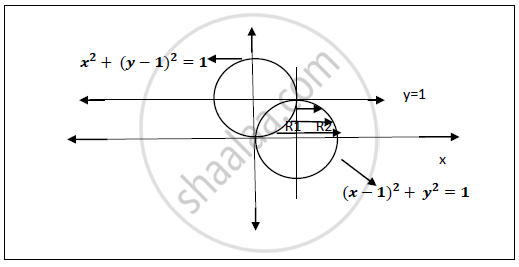Advertisement Remove all ads

# Change the Order of Integration of ∫ 1 0 ∫ 1 + √ 1 − Y 2 − √ 2 Y − Y 2 F ( X , Y ) D X D Y - Applied Mathematics 2

Change the order of integration of int_0^1int_(-sqrt(2y-y^2))^(1+sqrt(1-y^2)) f(x,y)dxdy

Advertisement Remove all ads

#### Solution

Let int_0^1int_(-sqrt(2y-y^2))^(1+sqrt(1-y^2)) f(x,y)dxdy

Region of integration : -sqrt(2y-y^2)≤ 𝒙 ≤ 𝟏+sqrt(1-y^2)

𝟎≤𝒚≤ 1

Curves : (i) x=-sqrt(2y-y^2) => x^2+y^2=2y  =>x^2+(y-1)^2=1

Circle with centre (0,1) and radius 1.

(ii) x=1+sqrt(1-y^2) =>  (x-1)^2+y^2=1

Circle with centre (1,0) and radius 1.

(iii) 𝒚=𝟎 𝒍𝒊𝒏𝒆 𝒊.𝒆 𝒆𝒒𝒖𝒂𝒕𝒊𝒐𝒏 𝒐𝒇 𝒙−𝒂𝒙𝒊𝒔.
(iv) 𝒚=𝟏 𝒍𝒊𝒏𝒆 𝒑𝒂𝒓𝒂𝒍𝒍𝒆𝒍 𝒕𝒐 𝒙−𝒂𝒙𝒊𝒔.Divide the region R into R1 and R2
∴ R = R1 ∪ R2
After changing the order of integration ,
For region R1 : 𝟎≤𝒚≤𝟏−sqrt(1-x^2)

𝟎≤𝒙≤𝟏
For region R2 : 0 ≤𝒚≤ sqrt(1-(x-1)^2)

𝟏 ≤ 𝒙 ≤ 𝟐

As the region is divided in two parts the integration will be the union of the two region limits.

"I"=int_0^1int_0^(1-sqrt(1-x^2)) f(x,y)dydx+int_1^2int_0^(sqrt(1-(x-1)^2))f(x,y)dydx

This is the integration after changing order from dx dy to dy dx of given integration region.

Concept: Change the Order of Integration
Is there an error in this question or solution?
Advertisement Remove all ads

#### APPEARS IN

Advertisement Remove all ads
Advertisement Remove all ads
Share
Notifications

View all notifications

Forgot password?